## V. Batyrev: “Toric Deformations of Some Spherical Fano Varieties”

Here are my (incomplete) notes from Batyrev’s talk in the Extremal Laurent Polynomials workshop at Imperial:

Batyrev_London_Sep_2010

If you have complete notes, please post them here.

Partial summary:

• spherical varieties
• a model family of examples: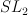$SL_2$-varieties
• review: compactification and toric varieties
• spherical compactification in these examples
• valuations and coloured cones
• finding toric degenerations

### One Comment

1.Sergey says:

Maybe it worth to note.

Yuri Prokhorov explianed to me that 2.21 with involution is actually a spherical variety – it has finite number of orbits with respect to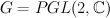$G = PGL(2,\mathbb{C})$.
Projective line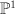$\PP^1$ is embedded by linear system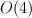$O(4)$ into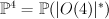$\PP^4 = \PP(|O(4)|^*)$,
and its automorphism group acts on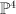$\PP^4$ preserving its image (twisted quartic C).